## Varying-coefficient SIRD model

In the post “A Tale of Two Cities”, I used a varying-coefficient SIRD model for curve fitting. In another post I gave a few technical details on model calibration by least-squares estimation of the parameters. I give a more detailed explanation in this post, and I also show how to obtain a faster (although less reliable) estimator of the reproduction number $$R(t)$$ using data smoothing.

The varying-coefficient SIRD model is as follows. In a population of size $$N$$, at a given time $$t$$ there are $$S(t)$$ susceptible individuals (not infected yet), $$I(t)$$ infectious individuals (who are infected and actively infecting others), $$R(t)$$ removed individuals (who are no longer infecting others, either because they recovered or isolated themselves), and $$D(t)$$ dead individuals. So $$S(t)+I(t)+R(t)+D(t)=N$$ at all times. The observable quantities are $$S(t)$$ and $$D(t)$$, which we can get from confirmed positive cases ($$S$$ would be $$N-P$$) and dead counts. The other two, $$I(t)$$ and $$R(t)$$, are not directly observable. Although some cities and countries report the number of recovered individuals, this is not the same as $$R(t)$$, because $$R(t)$$ includes, in addition to the recovered, those who are still sick but in isolation.

The model is given by the system of differential equations \begin{align} S'(t) &= - \beta(t) I(t) \frac{S(t)}{N}, \\ I'(t) &= \beta(t) I(t) \frac{S(t)}{N} - \gamma I(t) - \mu I(t), \\ R'(t) &= \gamma I(t), \\ D'(t) &= \mu I(t). \end{align}

Here we use a time-varying contact rate $$\beta(t)$$, obtaining then a time-varying reproduction number $$R(t)$$, $R(t) = \frac{\beta(t)}{\gamma + \mu}.$

## Estimating parameters by smoothing

Estimates of $$S(t)$$ and $$D(t)$$ can be obtained by smoothing the respective cumulative counts of positives and deaths ($$S$$ would be $$N-P$$). We use spline smoothers, although there are other possibilities (like kernel smoothers). For example, for the city of Buenos Aires we get the following (dots are the data, red lines are the smoothers):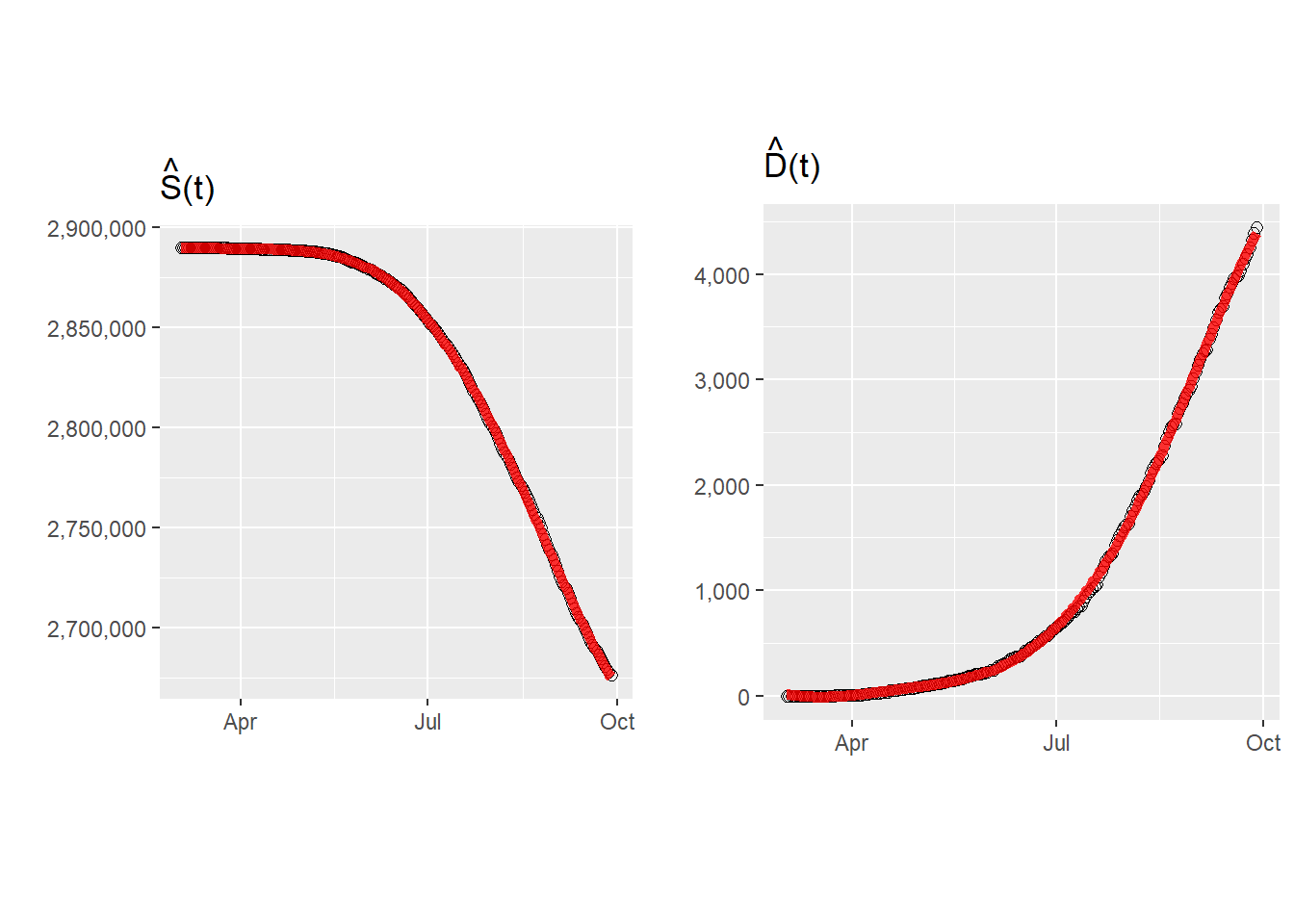We then obtain the derivatives of the spline smoothers, $$\hat S'(t)$$, $$\hat D'(t)$$ and $$\hat D''(t)$$: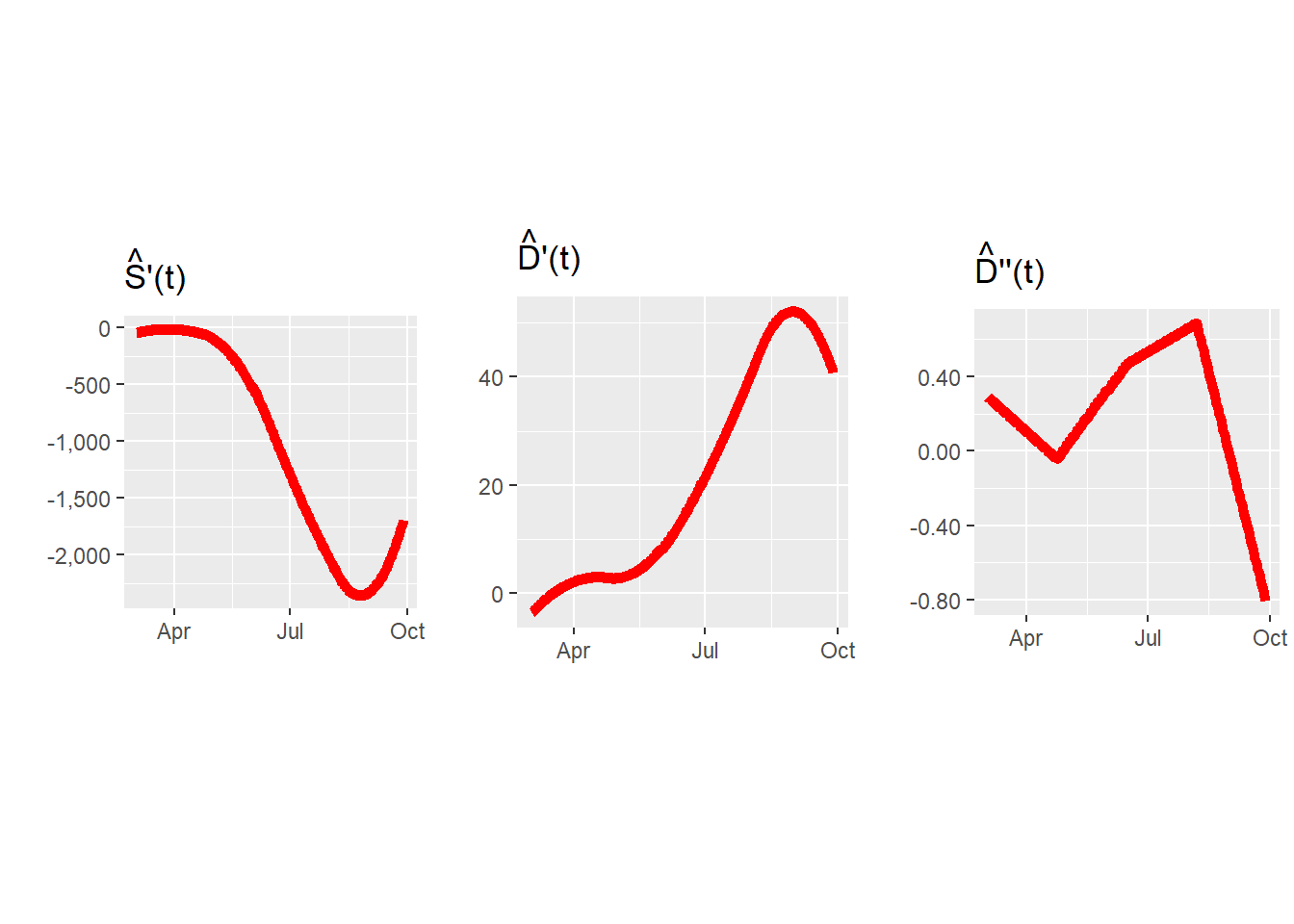An estimate of the active infecteds $$I(t)$$ can be obtained from the last equation of the SIRD model as $\hat I(t) = \frac{\hat D'(t)}{\hat\mu}.$ For this, we need an estimate of the mortality rate, $$\hat\mu$$. This can be obtained as the long term ratio of deads over positives. For Buenos Aires, the observed D/P ratio is: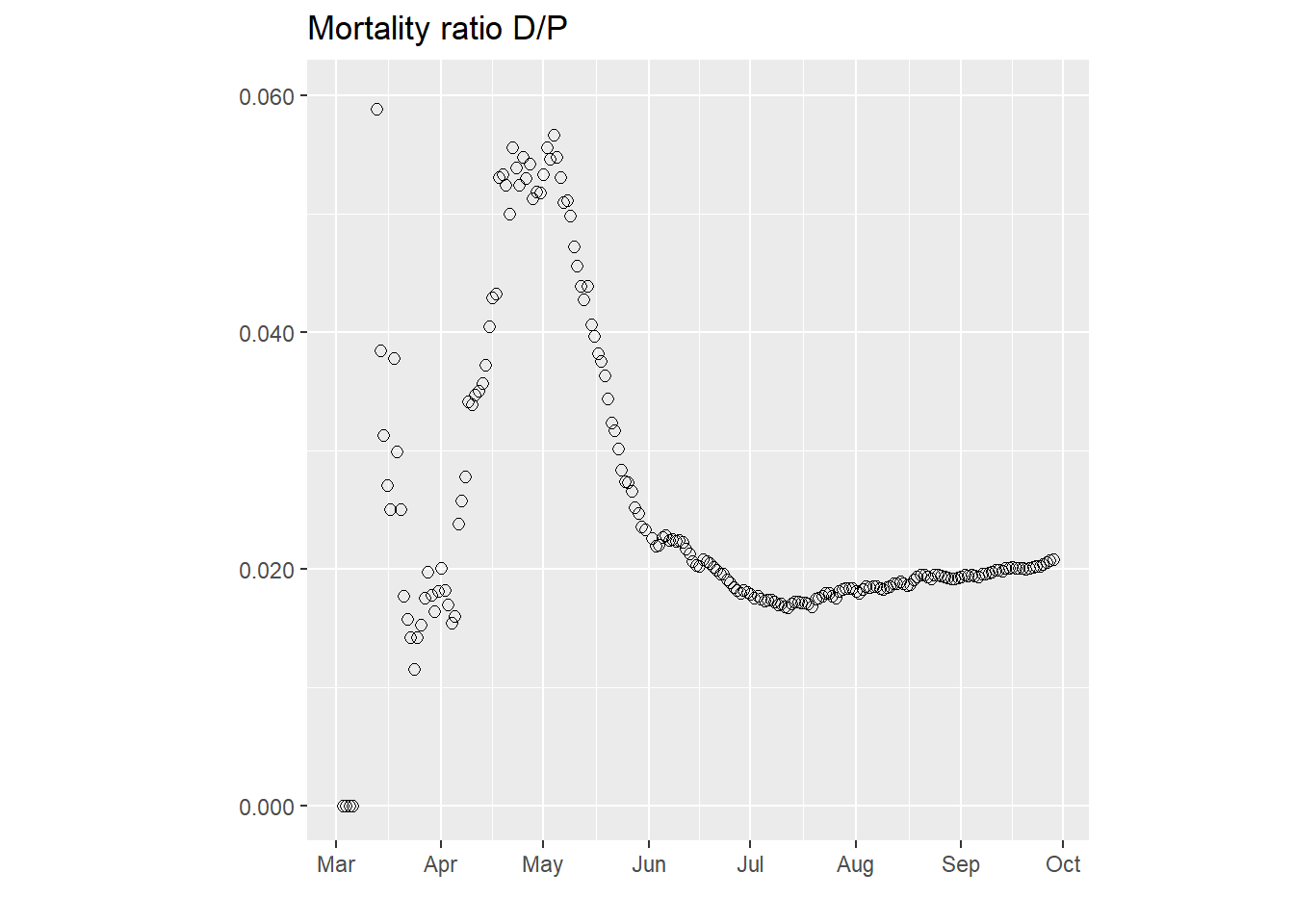After some initial variation, this curve stabilizes around 0.021, which we then take as $$\hat\mu$$. Then the estimated curve of active infecteds for Buenos Aires is: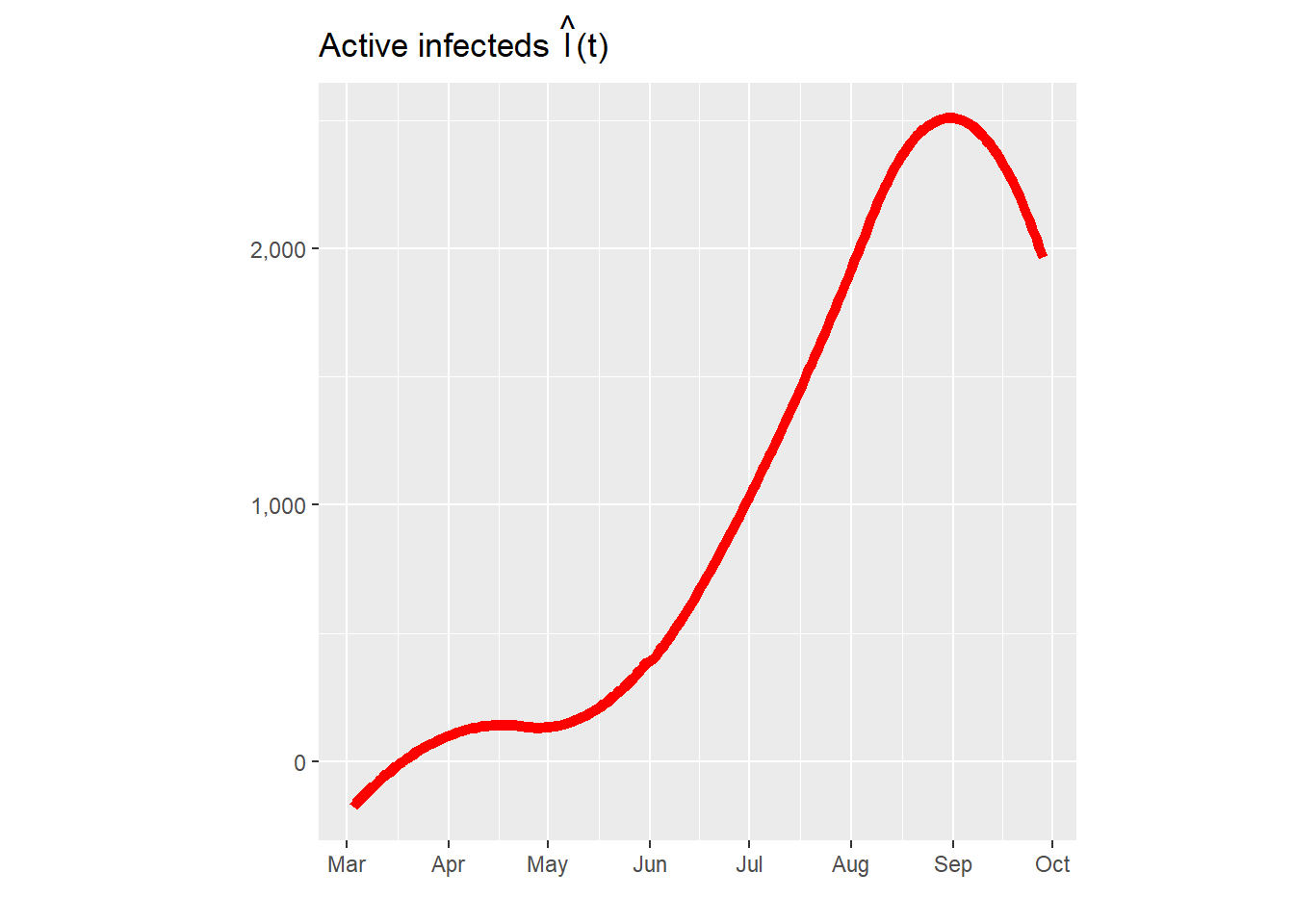Note that, due to border effects, estimation for the first few days is inaccurate and $$\hat I(t)$$ actually turns out negative.

Estimating the removal rate $$\gamma$$ is more complicated. From the second equation of the SIRD model, after substituting $$S'$$ and $$D'$$ from the first and fourth equations respectively, we get $\frac{D''(t)}{\mu} = -S'(t)-(\gamma + \mu)\frac{D'(t)}{\mu},$ so $\frac{-D''(t)-\mu S'(t)}{D'(t)} = \gamma + \mu.$ Then we can estimate $$\gamma+\mu$$ as the long-term value of the function on the left, which should be (in theory) approximately constant if the SIRD model is valid. For Buenos Aires we get: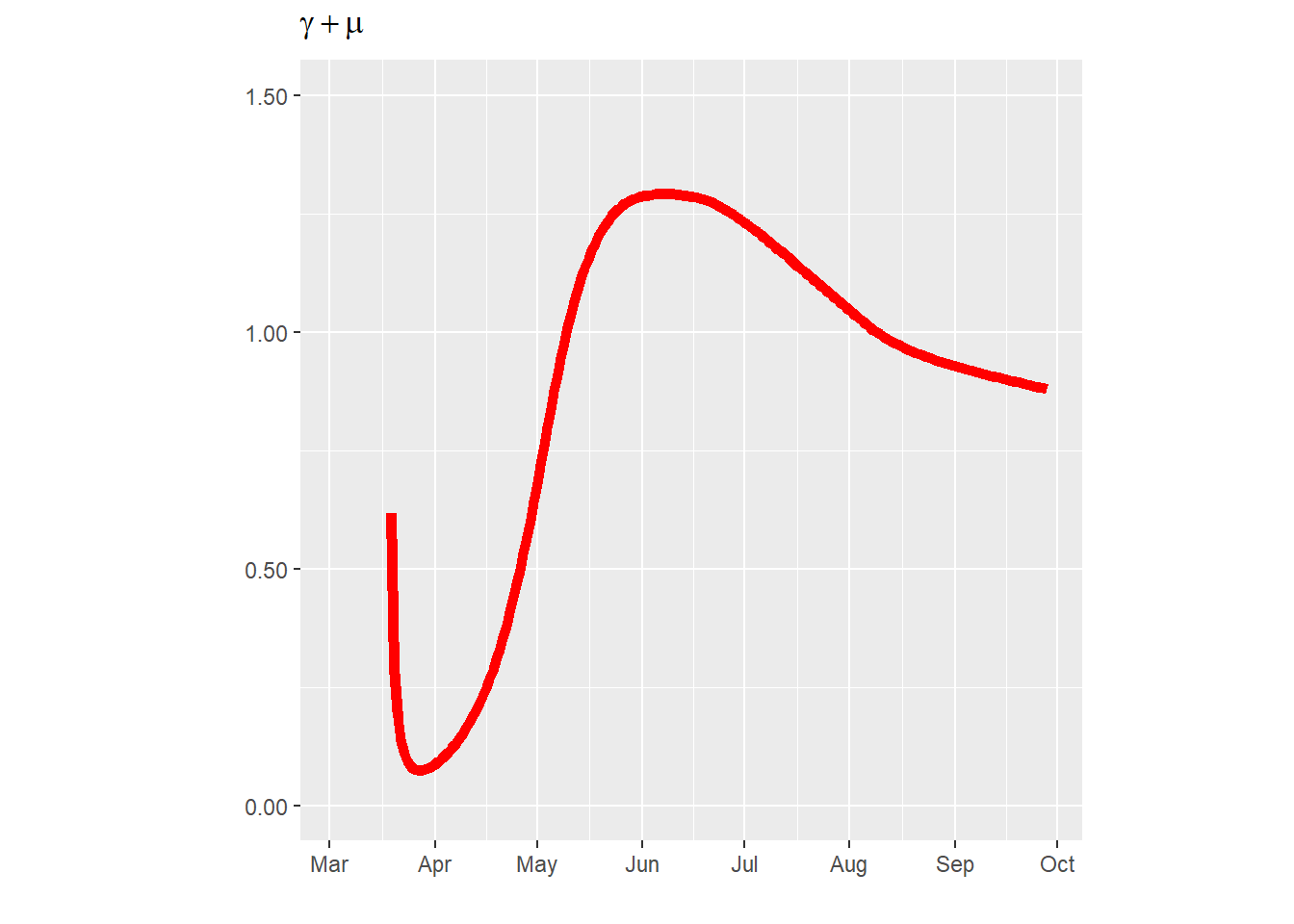After some initial variability, the ratio stabilizes around 0.879, so we take $$\hat \gamma$$ as 0.858.

An estimator of the contacts coefficient $$\beta(t)$$ is obtained from the first equation of the SIRD model as $\hat \beta(t) = \frac{-\hat S'(t)}{\hat I(t) \hat S(t)/N}.$ ($$\hat \beta(t)$$ is truncated at 0 if it turns out negative for some $$t$$s.) For Buenos Aires we obtain: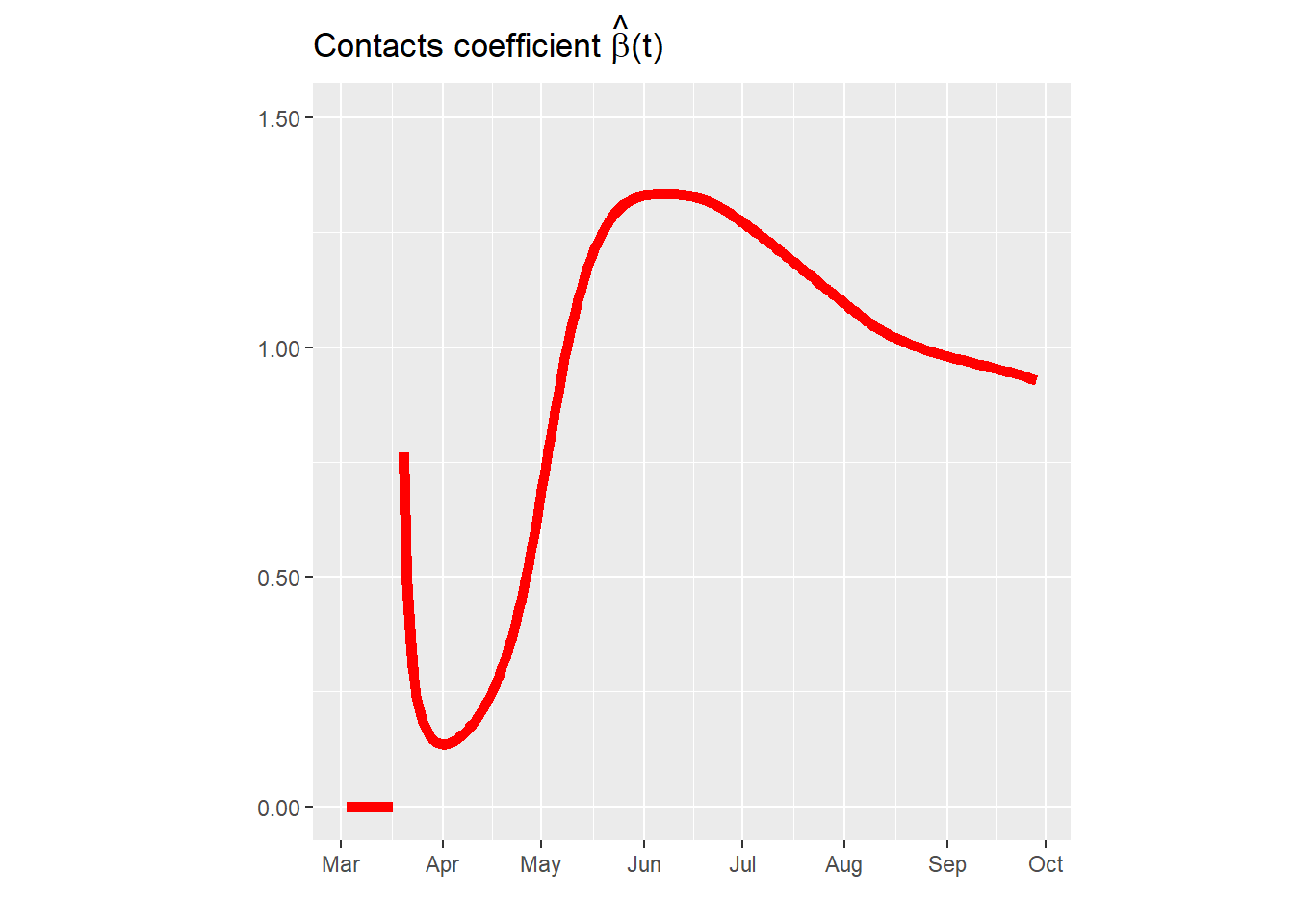Finally, putting everything together, we obtain the estimator of the time-varying reproduction number $$R(t)$$ as $\hat R(t) = \frac{\hat\beta(t)}{\hat\gamma + \hat\mu},$ which for Buenos Aires is: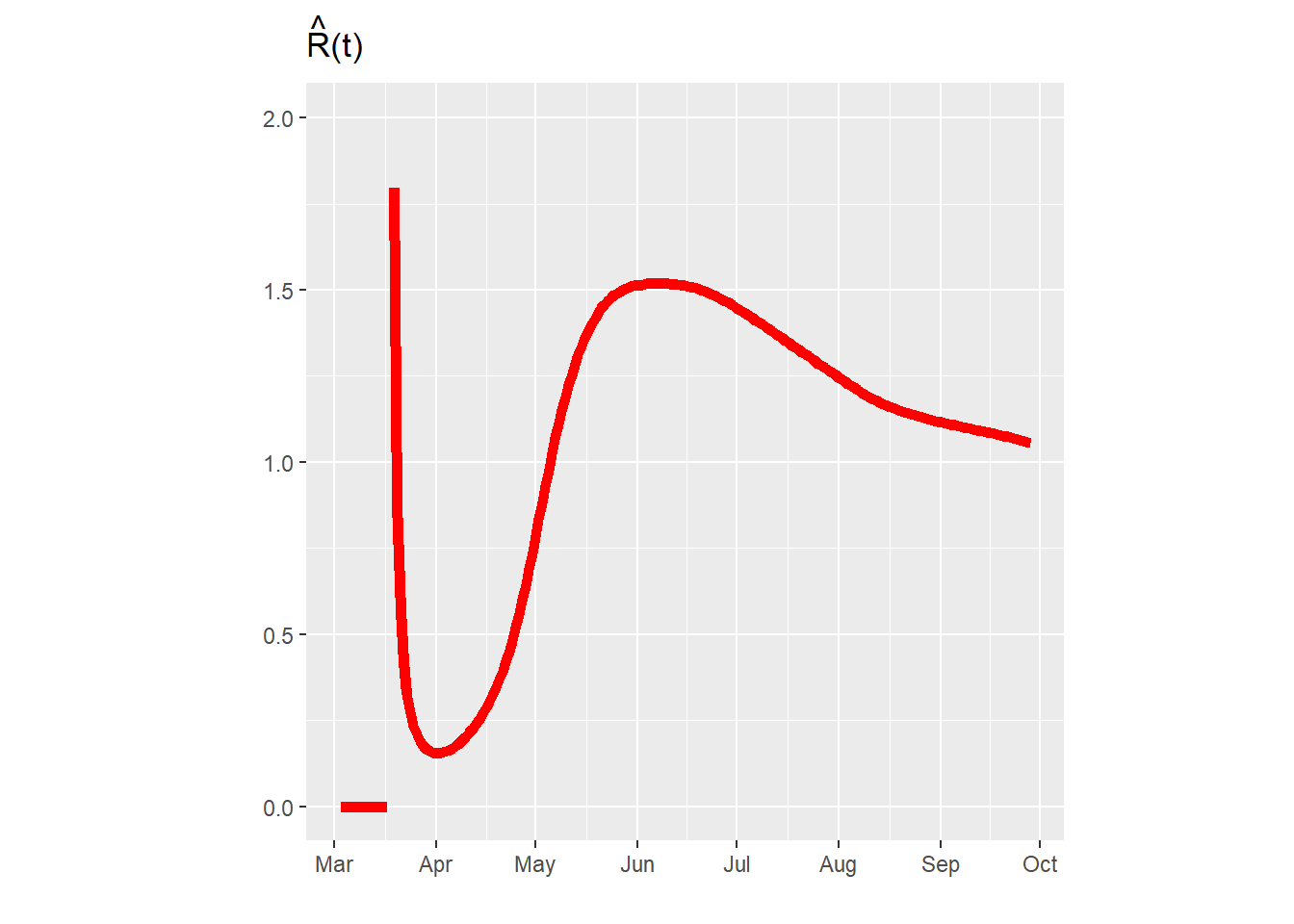This method provides a fast way to get rough estimates of $$R(t)$$, $$\mu$$ and $$\gamma$$, but we note that they have some shortcomings. In particular, if one feeds the above estimators $$\hat\beta(t)$$, $$\hat\mu$$ and $$\hat\gamma$$ into a differential-equation solver and computes $$S(t)$$ and $$D(t)$$, one does not get back the original smoothers $$\hat S(t)$$ and $$\hat D(t)$$, and sometimes there is a large discrepancy. Only the least-squares estimators explained below are consistent in this way.

## Estimating parameters by least squares

Better estimators, but computationally more expensive, can be obtained by least squares. If we denote by $$p_1,\ldots,p_n$$ the cumulative confirmed positives and by $$d_1,\ldots,d_n$$ the cumulative dead counts for days $$t_1,\ldots,t_n$$, and let $$s_i=N-p_i$$, then $\hat\theta = \arg \min \sum_{i=1}^n \{ \frac{(s_i-S(t_i,\theta))^2}{\sigma^2_s} + \frac{(d_i-D(t_i,\theta))^2}{\sigma^2_d} \},$ where $$\sigma^2_s$$ and $$\sigma^2_d$$ are the variances of the $$s_i$$s and $$d_i$$s, respectively. Here $$\theta$$ is the vector of parameters that include $$\mu$$, $$\gamma$$ and the spline coefficients of $$\beta(t)$$, which is modeled using spline function. More specifically, since $$\beta(t)$$ has to be non-negative we set $\beta(t) = \exp(\sum_{k=1}^q c_k \phi_k(t))$ where the $$\phi_k(t)$$s are spline basis functions. Similarly, since $$\gamma$$ and $$\mu$$ are constrained to $$(0,1)$$, we take their logit transforms to make them unconstrained, and then $\theta = (c_1,\ldots,c_q,\rm{logit}~\gamma,\rm{logit}~\mu)$ is a vector of unconstrained parameters.

The functions $$S(t,\theta)$$ and $$D(t,\theta)$$ are the ones obtained from the SIRD model using a differential-equation solver for a given $$\theta$$. Since the solver must be run for every update of $$\theta$$ of the minimization algorithm, the method is computationally more intensive than the one based on smoothing, but the estimators are more accurate and consistent.

For the city of Buenos Aires we get the following model fits (dots are the data, red lines are the SIRD fits):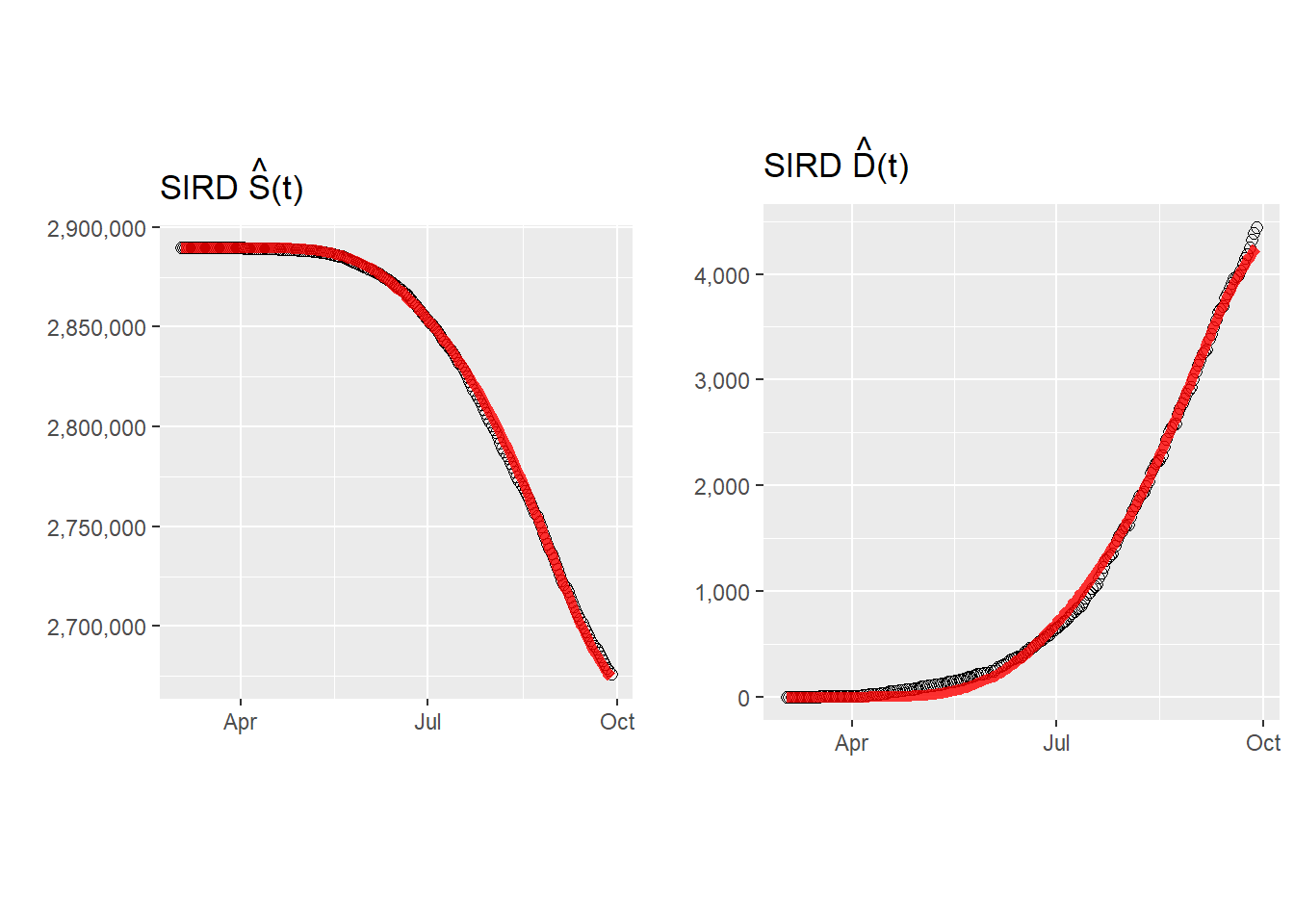And the following $$R(t)$$: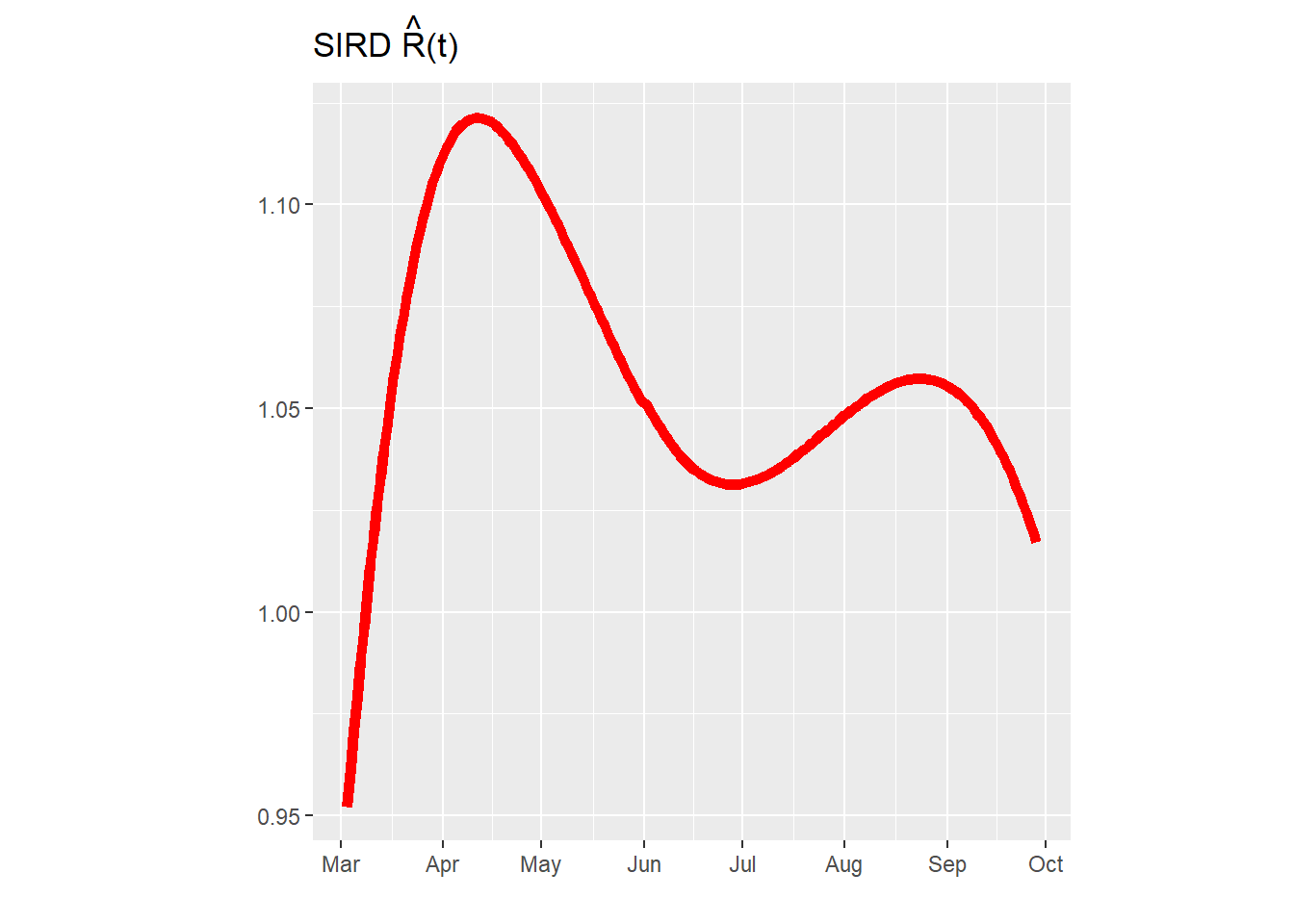This estimator of $$R(t)$$ is more stable than the one obtained from smoothers, especially for the earlier months. For the latter months both estimators are similar.

Back to blog